U.S. Department of Transportation
1200 New Jersey Avenue, SE
Washington, DC 20590
202-366-4000

Federal Highway Administration Research and Technology
Coordinating, Developing, and Delivering Highway Transportation InnovationsThis report is an archived publication and may contain dated technical, contact, and link information
 Federal Highway Administration > Publications > Research Publications > 04046 > Apph.Cfm Evaluation of Procedures for Quality Assurance Specifications
 Publication Number: FHWA-HRT-04-046 Date: October 2004

# Appenidx H: Distributionof Sample Pwl Estimates

### GENERATING SAMPLES

The plots in appendix H illustrate the distributions of sample PWL estimates for a number of different combinations of actual population PWL values. All of the plots are based on the same simulation results that came from simulating 1000 samples (sample size = 4) from a normal distribution with a mean of 0.00 and a standard deviation of 1.00. The sample means and standard deviations were then calculated for each of the 1000 samples. These values were then used to calculate sample PWL values for various actual population PWL values.

Figure 128 shows the distribution of the sample means for the 1000 samples. Statistical theory says that this should be a normal distribution with a mean equal to that of the population that was sampled (i.e., 0.00). Furthermore, the theory also says that the standard deviation of these 1000 sample means should be the population standard deviation (i.e., 1.00) divided by the square root of the sample size (i.e., 4 = 2 ). This means that the standard deviation of the sample means should be 0.50.

The average for the 1000 simulated sample means was +0.01, while their standard deviation was 0.484. These values are close to the theoretical ones and they are well within the range that might be expected from a simulation study. Figure 128 shows distribution histograms for the sample means and the sample standard deviations. As expected, the distribution of the sample means is approximately normal and is centered around the population mean of 0.00. Also, as expected, the distribution of the sample standard deviations is obviously skewed to the right. The average of the 1000 sample standard deviations is 0.941, which is close to the population value of 1.00.

### DISTRIBUTION OF SAMPLE PWL VALUES

The mean and standard deviation for each sample were used to estimate the PWL for populations with actual PWL values = 90, 80, 70, 60, and 50. For each population, both one-sided specifications and two-sided specifications were evaluated. For the two-sided specifications, the population mean was set in the middle of the specification range so that there were equal PD values for the lower and upper specification limits.

Figures 129 through 133 show the sample PWL distributions for both one-sided and two-sided populations with 90, 80, 70, 60, and 50 PWL, respectively. A trend regarding the shape of the distributions is apparent. For the population with its mean farthest from the specification limit(s) (i.e., the 90-PWL population), the distribution of the sample PWL values is skewed to the left. This is seen by the long tail to the left and the fact that about 60 percent of the distribution is to the right of the population PWL of 90. As the population mean moves closer to the specification limit(s) (i.e., moves from 80 to 70 to 60 to 50), skewness decreases until the distribution becomes symmetrical.

The reason that the sample PWL distributions are skewed stems from the fact that the distribution of the sample standard deviations is skewed. This skewness has less impact as the mean of the population approaches the specification limit. And, for a one-side specification to 384 have 50 PWL, the population mean must be centered on the specification limit. Any population that is centered on the one specification limit will have 50 PWL regardless of the value of its standard deviation. So, in this instance, the distribution of the sample PWL values will be symmetrical about the population mean. For any sample mean that is below the population mean, the estimated PWL will be less than 50, while any sample mean above the population mean will have an estimated PWL greater than 50. This is shown in figures 128 and 133 where both have 48.9 percent below and 51.1 percent above their population values.

Figure 134 shows diagrams of the populations that were sampled. These populations can be compared with the distributions of the sample PWL values shown in figures 129 through 133. For the one-sided specifications, the distribution whose mean is farthest from the specification limit (i.e., 90 PWL) has more PWL values above the true value than below it. As the distance between the population mean and the specification limit decreases, the distributions become symmetrical about the true population value.

For the two-sided specifications, once again, the population whose mean is farthest from the specification limits (i.e., 90 PWL) has more PWL values above the true value than below it. However, as the population means come closer to the specification limits (i.e., 60 PWL and 50 PWL), the distributions have more values below the true value than above it.

The two situations that were simulated (one-sided specifications and two-sided specifications, with the populations centered between the two limits) are the extremes with respect to how the PD for a population with a given PWL value can be distributed outside the two limits. There are obviously many other ways in which these PWL values could be located with respect to the specification limits. From these examples, it appears that the shape of the distribution of the sample PWL values will vary depending on how the PWL range of the population is located inside the limit or limits.

However, regardless of the shape of the distribution of the sample PWL values that were simulated, the average of the 1000 sample PWL values all differed from the population PWL value by no more than about 0.5 percent. Although the EP values were all very close for each of the distributions, the different shapes indicate that there may be small differences in the percentage of time that a population PWL value is estimated to be too high and too low, depending on the PWL of the population and where the population is centered with respect to the specification limit or limits.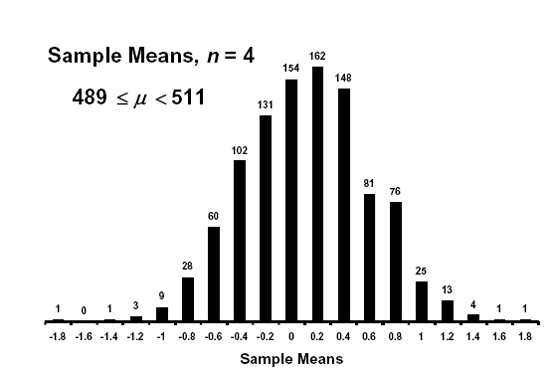Figure 128a. Distribution of sample means for 1000 samples from a normal population with μ = 0.00, σ = 1.00.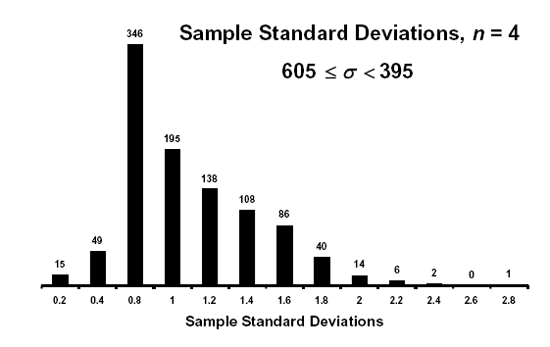Figure 128b. Distribution standard deviations for 1000 samples from a normal population with μ = 0.00, σ = 1.00.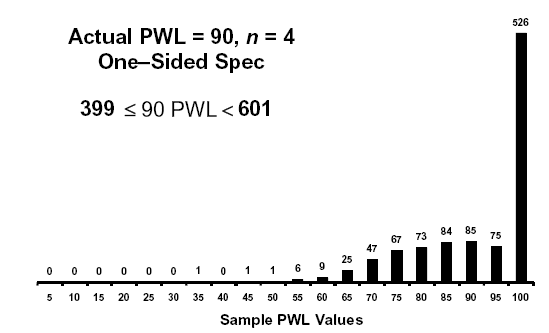Figure 129a. Distribution of sample PWL values for 1000 samples from a normal population with PWL = 90, one-sided specifications.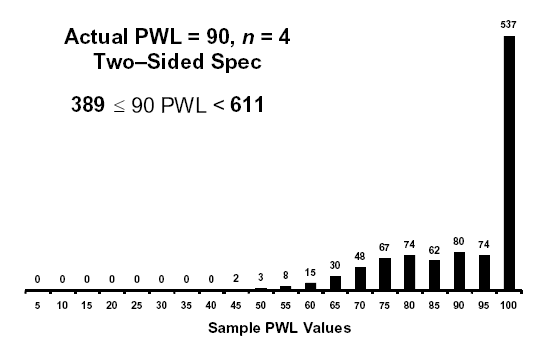Figure 129b. Distribution of sample PWL values for 1000 samples from a normal population with PWL = 90, two-sided specifications.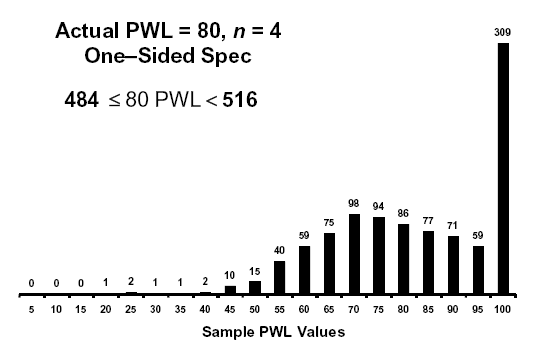Figure 130a. Distribution of sample PWL values for 1000 samples from a normal population with PWL = 90, one-sided specifications.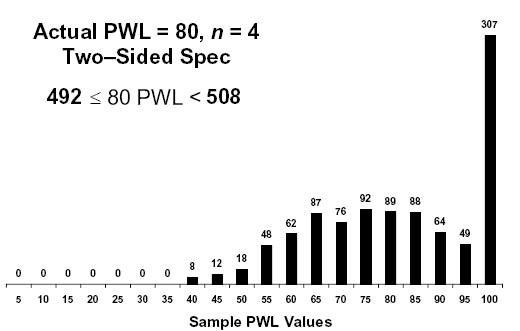Figure 130b. Distribution of sample PWL values for 1000 samples from a normal population with PWL = 80, two-sided specifications.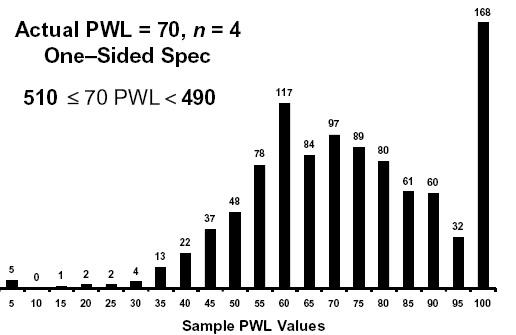Figure 131a. Distribution of sample PWL values for 1000 sample from a normal population with PWL = 80, one-sided specifications.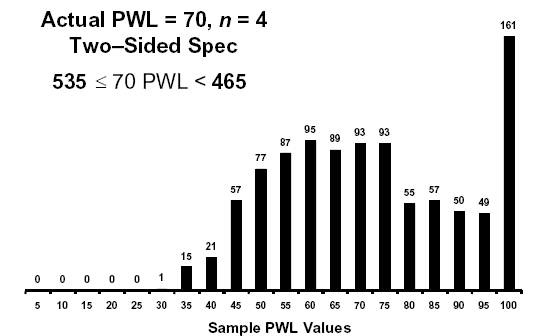Figure 131b. Distribution of sample PWL values for 1000 samples from a normal population with PWL = 80, two-sided specifications.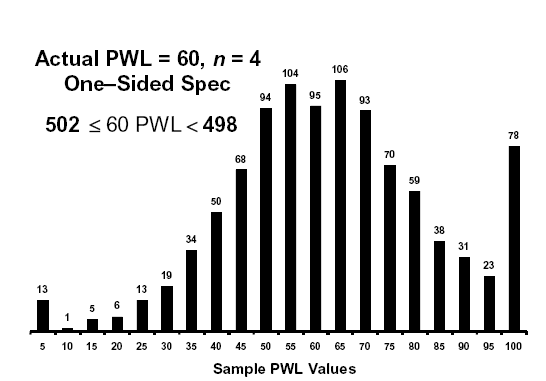Figure 132a. Distribution of sample PWL values for 1000 samples from a normal population with PWL = 60, one-sided specifications.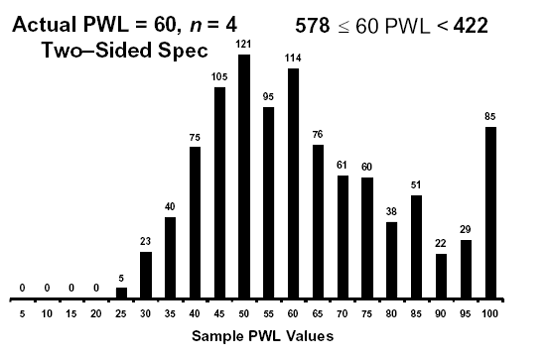Figure 132b. Distribution of sample PWL values for 1000 samples from a normal population with PWL = 60, two-sided specifications.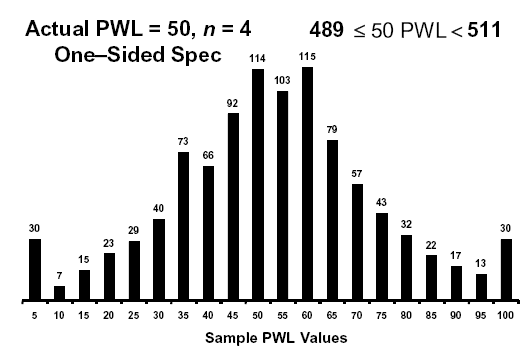Figure 133a. Distribution of sample PWL values for 1000 samples from a normal population with PWL = 50, one-sided specifications.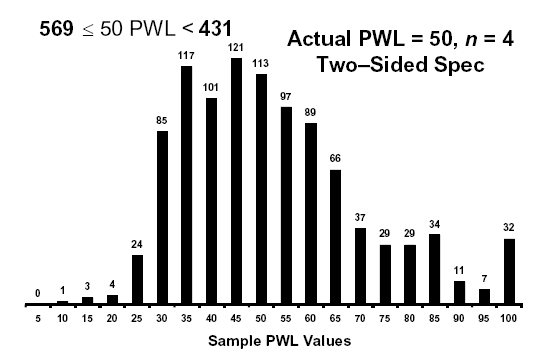Figure 133b. Distribution of sample PWL values for 1000 samples from a normal population with PWL = 50, two-sided specifications.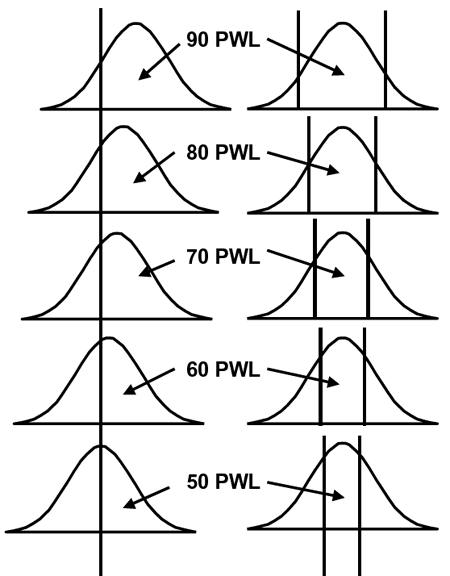Figure 134. Illustration of the populations for which the distributions of sample PWL values are shown in figures 129 through 133.